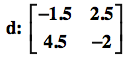Home > A2C > Chapter 9 > Lesson 9.2.2 > Problem9-97

9-97.
1. Given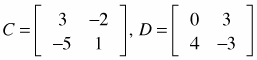find: Homework Help ✎

1. CD

2. C + D

3. C2

4. X if 2X + C = D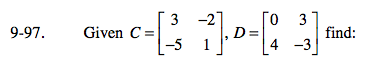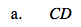Multiply each row of the first matrix into each column of the second matrix.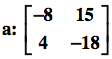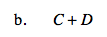Add the numbers in the corresponding entries.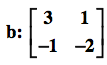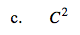C 2 = C · C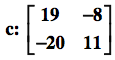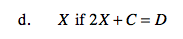2X + C = D
2X = D − C

$\textit{X}= \frac{(\textit{D}-\textit{C})}{2}$